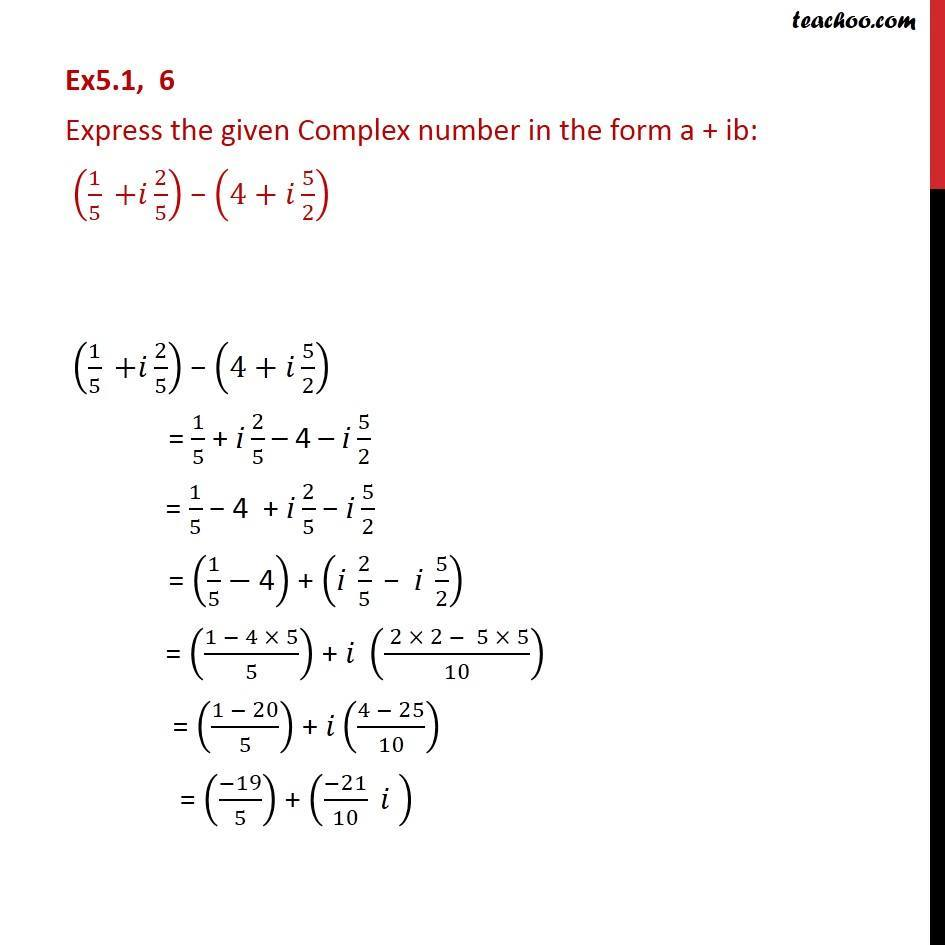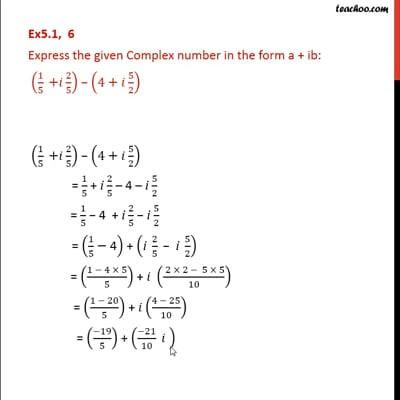Ex 5.1

Chapter 5 Class 11 Complex Numbers (Term 1)
Serial order wiseThis video is only available for Teachoo black users

### Transcript

Ex5.1, 6 Express the given Complex number in the form a + ib: (1/5 "+" 𝑖 2/5) − (4+𝑖 5/2) (1/5 "+" 𝑖 2/5) − (4+𝑖 5/2) = 1/5 + i 2/5 – 4 – i 5/2 = 1/5 − 4 + i 2/5 − i 5/2 = (1/5−"4" ) + ("i" 2/5 " − i " 5/2) = ((1 − 4 × 5)/5) + 𝑖 (( 2 × 2 − 5 × 5)/10) = ((1 − 20)/5) + 𝑖((4 − 25)/10) = ((−19)/5) + ((−21)/10 𝑖 )